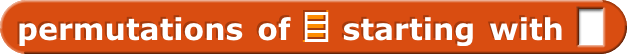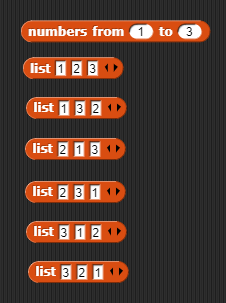# Number Pattern Finder

Hello! I have made a system that attempts to find all patterns in an arrangement of numbers. For example, I could have 12345, 54321, 13542, or 24531. I have also made a code-cracking block that can give you *maybe give you all password from up to 9 digits long. Here is how this system works:

1. Create arrangement of numbers into a list
2. Replace each item in the list with a random number
3. Compress the list into a single number
4. Check if the number has already been found
5. (If number has not been found yet) add number to a seperate list

If your still confused, here is an image:

If you want to check this out, here is the link:

Feel free to give suggestions!

Umm. Random guessing isn't a good way to find all the possibilities. The number of rearrangements of n values is n!, because first you pick one of the values (n possibilities), then you pick one of the remaining n-1 values, then one of the remaining... etc. So in your five-values example, there are only 5! = 120 arrangements, and in 1000 guesses you're likely to find a lot of them even though some will be duplicates. But 10! = 3628800, so it'd take millions of guesses.

The official textbook way to find all the permutations (that's the technical term for "rearrangements") of a set of values is to divide that set of permutations, in your head before writing any code, into those that start with the first value and those that don't start with the first value. Then figure out how to list all the permutations of each kind, using recursion to solve a smaller problem.

In other words, write this helper function:It checks if its already been found
Edit: I figured out it could be that snap is only displaying 100 numbers, so the program is probqbly only check that 100 problems.

If this is how to find the amount of permutations, how would I be able to do that if you dont know how many permutations you have?

Im not very good at math, so I dont quite understand how you explained it to me.

Ok, so I read up on it, and using permutations is basically changing the first number to a random number, but we can no longer use that number. So for the second number, we decide a random number without using the number we already used. Then the third, choose random number without using already used numbers, and so on. Am I right?

not quite - a number can appear in a position several times e.g

This shows all the permutations of 1,2,3I think he meant, in a single permutation you can't reuse a number.

I know; I wasn't saying there'd be duplicates in the final result, just that if you make 1000 guesses that doesn't mean you'll get 1000 unique answers.

Finding the number of permutations is easy; it's n factorial. We're talking about finding the actual permutations. What I was trying to say, to take a very simple example, is that if you're looking for permutations of (1, 2, 3) you mentally divide them into the ones that start with 1 (two of them, (1, 2, 3) and (1, 3, 2)) and the ones that don't start with 1 (four of those). Then write a Snap! expression that reports each of those groups, and use APPEND to get the complete result.

I'm trying to explain this without actually writing the code for you, which would kind of ruin the fun.